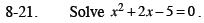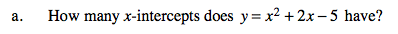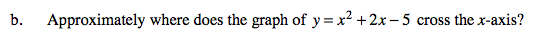### Home > CCA2 > Chapter 8 > Lesson 8.1.1 > Problem8-21

8-21.
1. Solve x2 + 2x − 5 = 0. Homework Help ✎

1. How many x-intercepts does y = x2 + 2x − 5 have?

2. Approximately where does the graph of y = x2 + 2x − 5 cross the x-axis?You could complete the square, find the vertex, and consider the orientation to determine if this parabola has 0, 1, or 2 x-intercepts.